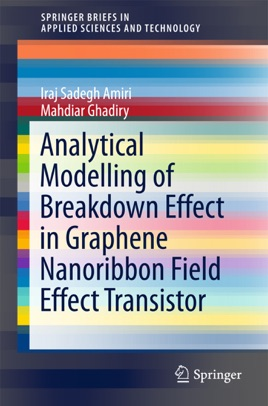• 46,99 €

## Beschreibung des Verlags

This book discusses analytical approaches and modeling of the breakdown voltage (BV) effects on graphene-based transistors. It presents semi-analytical models for lateral electric field, length of velocity saturation region (LVSR), ionization coefficient (α), and breakdown voltage (BV) of single and double-gate graphene nanoribbon field effect transistors (GNRFETs). The application of Gauss’s law at drain and source regions is employed in order to derive surface potential and lateral electric field equations. LVSR is then calculated as a solution of surface potential at saturation condition. The ionization coefficient is modelled and calculated by deriving equations for probability of collisions in ballistic and drift modes based on the lucky drift theory of ionization. The threshold energy of ionization is computed using simulation and an empirical equation is derived semi-analytically. Lastly avalanche breakdown condition is employed to calculate the lateral BV. On the basis of this, simple analytical and semi-analytical models are proposed for the LVSR and BV, which could be used in the design and optimization of semiconductor devices and sensors. The proposed equations are used to examine BV at different channel lengths, supply voltages, oxide thickness, GNR widths, and gate voltages. Simulation results show that the operating voltage of FETs could be as low as 0.25 V in order to prevent breakdown. However, after optimization, it can go as high as 1.5 V. This work is useful for researchers working in the area of graphene nanoribbon-based transistors.